## ACT test prepthat actually works

### November 2018

#### Solution PageWe at 36U want to thank the many students and teachers who participated in our November 2018 Winter Challenge. We hope you enjoyed working the item and learned a new skill or two along the way!

For those who missed it, here was the challenge problem:

The Problem

In a fountain, there are 1,000 quarters, 2,000 dimes, 2,000 nickels, and 5,000 pennies. A student randomly draws a coin from the fountain each day for 3 years, records its value, and tosses it back into the fountain.

Let x equal the value of a coin drawn randomly from the fountain. If all coins are equally likely to be drawn, regardless of coin size, what is the expected value (long-run average) of x?

A. 1¢

B. 4¢

C. 6¢

D. 8¢

E. 10¢

The Solution

The expected value, or long-run average, of coins drawn randomly from the fountain is 6 cents.

Almost every submission was correct. Great work!

Here’s how we worked the item:

Step 1: Find the probability of each outcome.

The first step in solving expected value items is to find the probability of each outcome. Often, the ACT will provide those probabilities in a probability distribution table.

Finding the probabilities will allow us to weight each outcome in Step 2.

Here’s the probability of each outcome:

P(penny) = 5,000 pennies/10,000 total coins = 1/2

P(nickel) = 2,000 nickels/10,000 total coins = 1/5

P(dime) = 2,000 dimes/10,000 total coins = 1/5

P(quarter) = 1,000 quarters/10,000 total coins = 1/10

Step 2: Weight each outcome.

Now that you know the probability of each outcome, weight each outcome value by multiplying by its probability.

One-half of the time, the student draws a penny that is worth 1 cent.

1/2 •  1¢ –> .5¢

One-fifth of the time, the student draws a nickel that is worth 5 cents.

1/5 •  5¢ –> 1¢

One-fifth of the time, the student draws a dime that is worth 10 cents.

1/5 •  10¢ –> 2¢

One-tenth of the time, the student draws a quarter that is worth 25 cents.

1/10 •  25¢ –> 2.5¢

The probabilities add to 1. When we multiplied the probabilites by the values, we essentially broke down a single draw from the fountain into all the possible outcomes, weighted, of course.

Now it’s time to add those values to see what an average draw from the fountain would give.

Expected value = .5¢ + 1¢ + 1¢ + 2.5¢

Expected value = 6¢

The expected value, or long-run average, of a coin drawn randomly from the fountain is 6¢.

Impressive solution!

One of our favorite problem solving approaches came via Facebook. Check it out.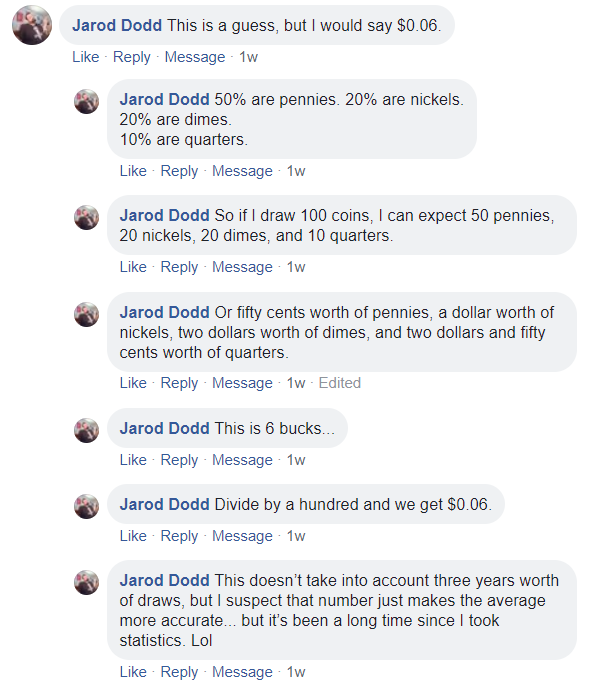The Winner

Congratulations to Bailey S., our November 2018 Expected ValueChallenge winner! Her solution was simple, precise, and just as important, correct. Here’s what she had to say: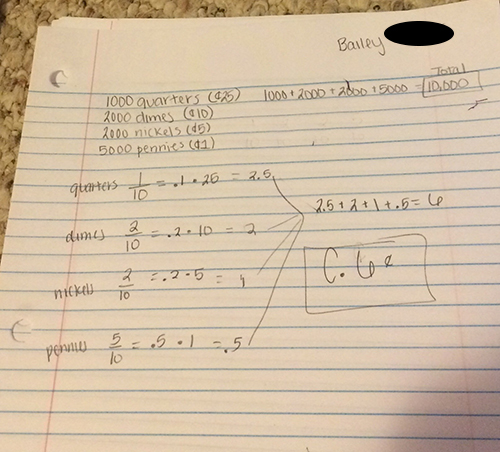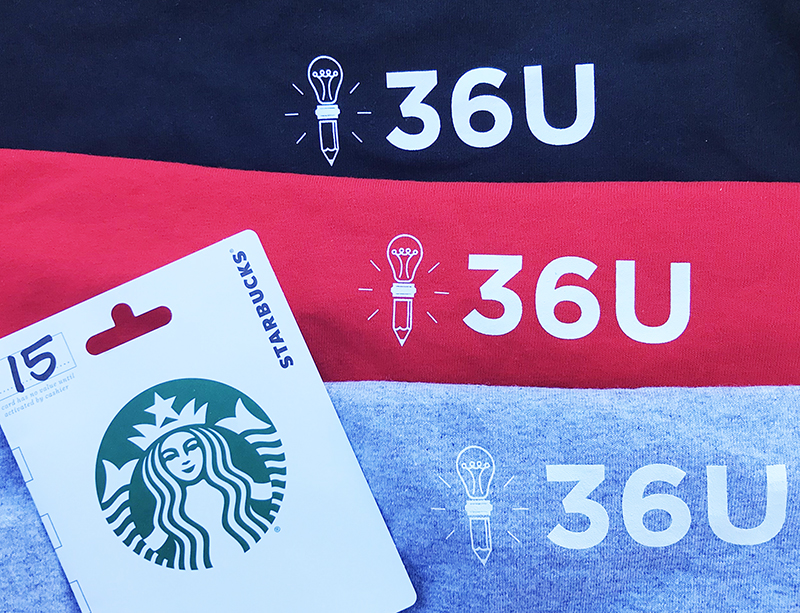The Winnings!

Bailey won the 36U Winter Care Package:

36U Tee (Long-sleeve)

### Expected Value Challenge Item Giveaway

#### November 2018

Statistics and probability are a new focus for the ACT.  We at 36U have come up with a fun challenge to help you prepare for this new focus area–AND to give you a chance to win prizes!

In a fountain, there are 1,000 quarters, 2,000 dimes, 2,000 nickels, and 5,000 pennies. A student randomly draws a coin from the fountain each day for 3 years, records its value, and tosses it back into the fountain.

Let x equal the value of a coin drawn randomly from the fountain. If all coins are equally likely to be drawn, regardless of coin size, what is the expected value (long-run average) of x?

A. 1¢

B. 4¢

C. 6¢

D. 8¢

E. 10¢

How Do I Enter the Contest?

Take a pic of your solution, with your work included, and post as a reply to any of our Expected Value Fountain Challenge social media posts OR email to support@36university.com. Submissions must be posted by midnight eastern time on November 15th. Impress us with your easily-understood, clear solution!What Will I Win?

The winner will receive the 36U Winter Care Package:

36U Tee (Long-sleeve)

How Will 36U Choose a Winner?

At 36U, we value simple, precise solutions. We will draw a winner on November 16th from among entries with correct answers and easy-to-understand explanations. The winner’s submission will be included in  our solution post.

Post Follow-up: Working the New ACT Math Probability Items

In the previous post, we divided the probability items found in the last two Preparing-for-the-ACT Guides into three categories. In this post, we work those items. If you have questions or comments, shoot me an email at kshipley@36university.com.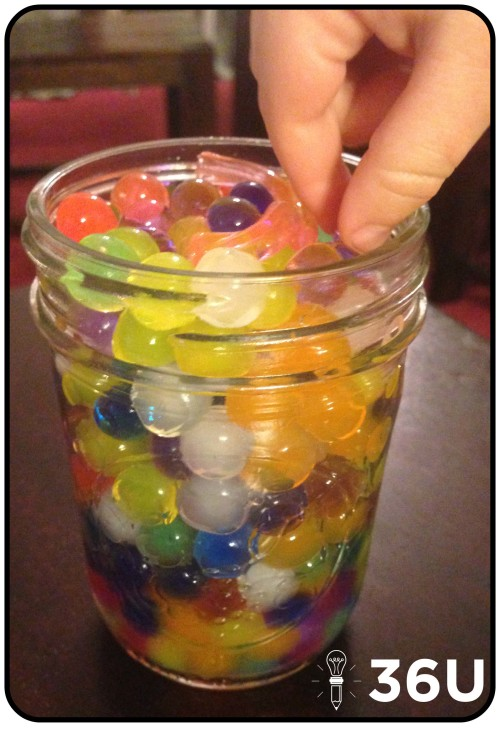These items are provided to give you examples of the ways you can expect ACT to test probability. These are screenshots from the ACT’s booklets, not the property of 36 University.

Item Type 1: Basic Probability Items

These items require you to apply the basic probability formula:

P(A) = (# of events corresponding to A/ total # of possible events)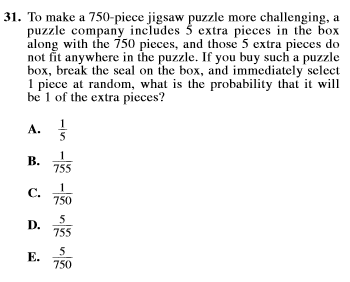#31 Solution:

Step 1) Recognize there are now 755 pieces.

Step 2) Of those 775 pieces, 5 are the new “extra” pieces.

Step 3) Use probability formula: P(extra piece) = 5/755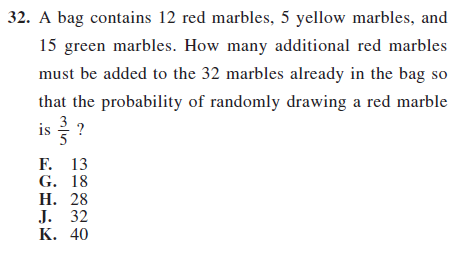#32 Solution:

Step 1) Current probability of red marble: 12/32

Step 2) Recognize we need a fraction that will reduce to 3/5, so the denominator has to reduce to 5.

Step 3) Begin adding red marbles in increments so that the total number of red marbles is divisible by 3.

Current Probability of red: 12/32 –> 3/8

Add 3 red marbles. Updated probability of drawing red marble: 15/35 –> 3/7

Add 8 red marbles. Updated probability of drawing red marble: 20/40 –> 1/2

Add 13 red marbles. Updated probability of drawing red marble: 25/45 –> 5/9

Add 18 red marbles. Updated probability of drawing red marble: 30/50 –> 3/5.  (I should have known!)

Item Type 2: Probability from Graphs

These items require you to utilize your graph reading skills and basic probability concepts.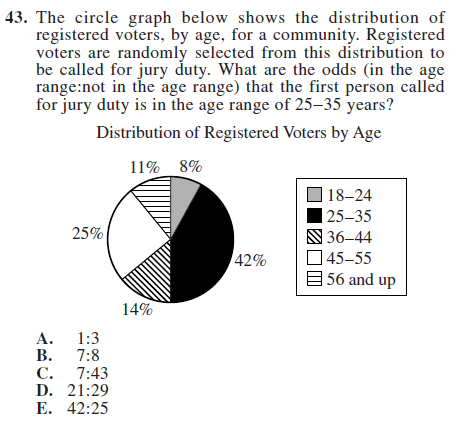#43 Solution:

Step 1) They provide formula for odds. Odds = in the age range:not in the age range.

Step 2) Percentage of persons in 25-35 years range: 42% or 42 out of 100.

Step 3) If 42 out of 100 are in the age range, the other 58 must not be in the age range.

Step 4) Set up the odds. 42:58 –> 21:29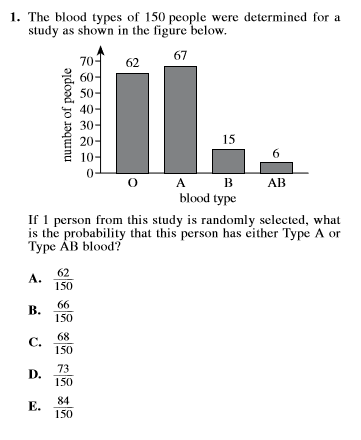#1 Solution:

Step 1: P(Type A or Type AB blood) = (# of Type A + # of Type AB)/ (Total # of possibilities)

Step 2: P(Type A or Type AB blood) = (67 + 6)/150 –> 73/150

Item Type 3: Probability of Multiple Events

Multiple events probability items ask you take one additional step. Often, that means you’ll just need to multiply the probabilities of each event. Here are examples: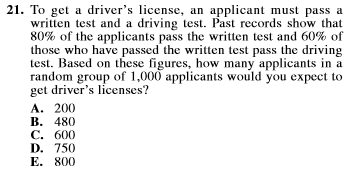#21 Solution:

Step 1: 80% pass written test. Of 1,000, we expect 800 to pass written test. (80% • 1000)

Step 2: Of those that pass written test, 60% pass driving test. 60% • 800 that passed written –> 480 expected to pass both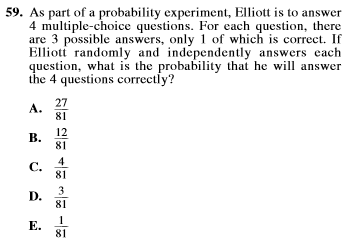#59 Solution:

Step 1: Chances of guessing a particular item correctly is 1/3, since there are three answer choices.

Step 2: Chances of guessing all 4 items correctly? Multiply the probabilities: (1/3) • (1/3) • (1/3) • (1/3) –> 1/81

Wrapping It Up

We hope these solutions helped you work these items more efficiently! If you have an alternative, quicker method to solving any of these items, we’d love to see it.

Recent & Related Posts:

3 Types of Probability Items to Expect on the ACT Math Test

Origami: Sharpen Visual-Spatial Skills & Boost Your ACT Math Score

Your Week-Before-the-ACT Game Plan (Part 4 of 4: Science)

36U ACT Tips

36U Blog List

36U ACT Prep Program

Three Types of Probability Items to Expect on the ACT Math Test

You walk into the testing site and sit down, nervous but confident. You know you’ve prepared and you’re ready to give the ACT one last shot. You are sure this will result in your best your score yet. The English test is no problem. You open the math test and begin working, but something has changed…Modifications to the ACT Math Test

Maybe you haven’t heard, but ACT announced test-takers could look forward to “the inclusion of additional statistics and probability items in the mathematics test” (Source: ACT FAQ). ACT hasn’t released volumes of materials on the changes, but the last two released exams contained in the Preparing-for-the-ACT Guides are consistent with the announced changes and provide the best clues we have on the amendments. We have divided the following items into three categories. The items are provided to give you examples of the ways you can expect ACT to test probability. These are screenshots from the ACT’s booklets, not the property of 36 University.

Item Type 1: Basic Probability Items

These items require you to apply the basic probability formula:

P(A) = (# of events corresponding to A/ total # of possible events)Item Type 2: Probability from Graphs

These items require you to utilize your graph reading skills and basic probability concepts.Item Type 3: Probability of Multiple Events

Multiple events probability items ask you take one additional step. Often, that means you’ll just need to multiply the probabilities of each event. Here are examples:Wrapping It Up

The good news is these items aren’t that complicated and are likely to replace more difficult items. These changes may mean it’s time for you to brush up on basic probability, especially since ACT may begin testing these concepts in more challenging ways.

Do you want to see these items worked? Check out our follow-up post. For more practice, check out the online Sample Items published by ACT. Specifically, try Set 3 Item #2.

Recent & Related Posts:

Post Follow-up: Working the New ACT Math Probability Items

Origami: Sharpen Visual-Spatial Skills & Boost Your ACT Math Score

Your Week-Before-the-ACT Game Plan (Part 4 of 4: Science)